# Lions Weekly: January 11, 2023# A Note From Mr. Sandefur

Lion Family,

It has been a great start to the second week of this semester. As many events are scheduled for this semester, I want to take time to encourage you to join us on campus.

I would also like to personally take the time to encourage you to consider re-enrolling your student during our priority re-enrollment window (until February 3rd) if you have not already done so. By enrolling through this process, our leadership team is able to plan accordingly and take the necessary steps for the next school year. If you have any questions regarding the re-enrollment process, our admissions office or I would be more than willing to assist in any way possible.

As always, please know we are thankful for each and every one of you and are praying that you have a blessed and beneficial week!

Jeremy Sandefur

 table div table+table+table+table div table{width:100%;padding:0}table div table+table+table+table div table img{width:96.23%;padding:0;float:none}table div table+table+table+table div table td{width:100%;padding:0 1.88% 18px}/* styles */table div table+table+table+table+table+table div table{width:100%;padding:0}table div table+table+table+table+table+table div table img{width:96.23%;padding:0;float:none}table div table+table+table+table+table+table div table td{width:100%;padding:0 1.88% 18px}/* styles */# Monday, January 16

## School Closed

On Monday, January 16, TKA school and offices will be closed as we take time to honor and remember Dr. Martin Luther King, Jr.

 table div table+table+table+table+table+table+table+table div table{width:100%;padding:0}table div table+table+table+table+table+table+table+table div table img{width:96.23%;padding:0;float:none}table div table+table+table+table+table+table+table+table div table td{width:100%;padding:0 1.88% 18px}/* styles */# Priority Re-enrollment is Now Open

We hope that 2023 is off to a wonderful start as we look forward to all that God has in store for The King’s Academy in the coming months. Thank you for the privilege of partnering with you to equip your child to impact the world for Christ!

Re-enrollment for the 2023-2024 school year is now open! To assist our administrative team in planning for the coming year, it is vital that all current students re-enroll as soon as possible. As an incentive, we are offering an early enrollment discount to everyone who submits re-enrollment through February 3. The regular re-enrollment fee of \$300 per student will be reduced to only \$100 per student if re-enrollment is completed by February 3, 2023!
Click the following link to complete your re-enrollment form and make payment:

Please note that re-enrollment CANNOT be completed on a smartphone or tablet.

## Instructions for completing re-enrollment:

Please note that this form looks different than previous years as we are working to streamline this process as much as possible.

• After logging in, click on the “Apply/Enroll” tab on the left, then click “Enrollment/Reenrollment.”

• Follow the instructions on the screen to complete the re-enrollment packet. Please be sure to update any information that has changed over the past year such as address, insurance, and phone numbers.

• Once all information is updated and complete, FACTS will ask for the re-enrollment fee.

Please remember that re-enrollment is for current TKA students only. Any siblings of current students or new students who have not been enrolled at our school prior to this point will need to start an application found on our website.

Contact the Admissions and Enrollment Office by phone or email if you have any questions or need help with the re-enrollment process. We hope to see all of our families return to TKA next year! We are thankful for the opportunity to partner with you in the education and development of your students.

Sincerely,

Lori Campbell
(865) 573-8321 option 1

## Pertinent information regarding the 2023-24 academic year can be found by utilizing the below listed links:

 table div table+table+table+table+table+table+table+table+table+table+table+table+table+table+table+table div table{width:100%;padding:0}table div table+table+table+table+table+table+table+table+table+table+table+table+table+table+table+table div table img{width:96.23%;padding:0;float:none}table div table+table+table+table+table+table+table+table+table+table+table+table+table+table+table+table div table td{width:100%;padding:0 1.88% 18px}/* styles */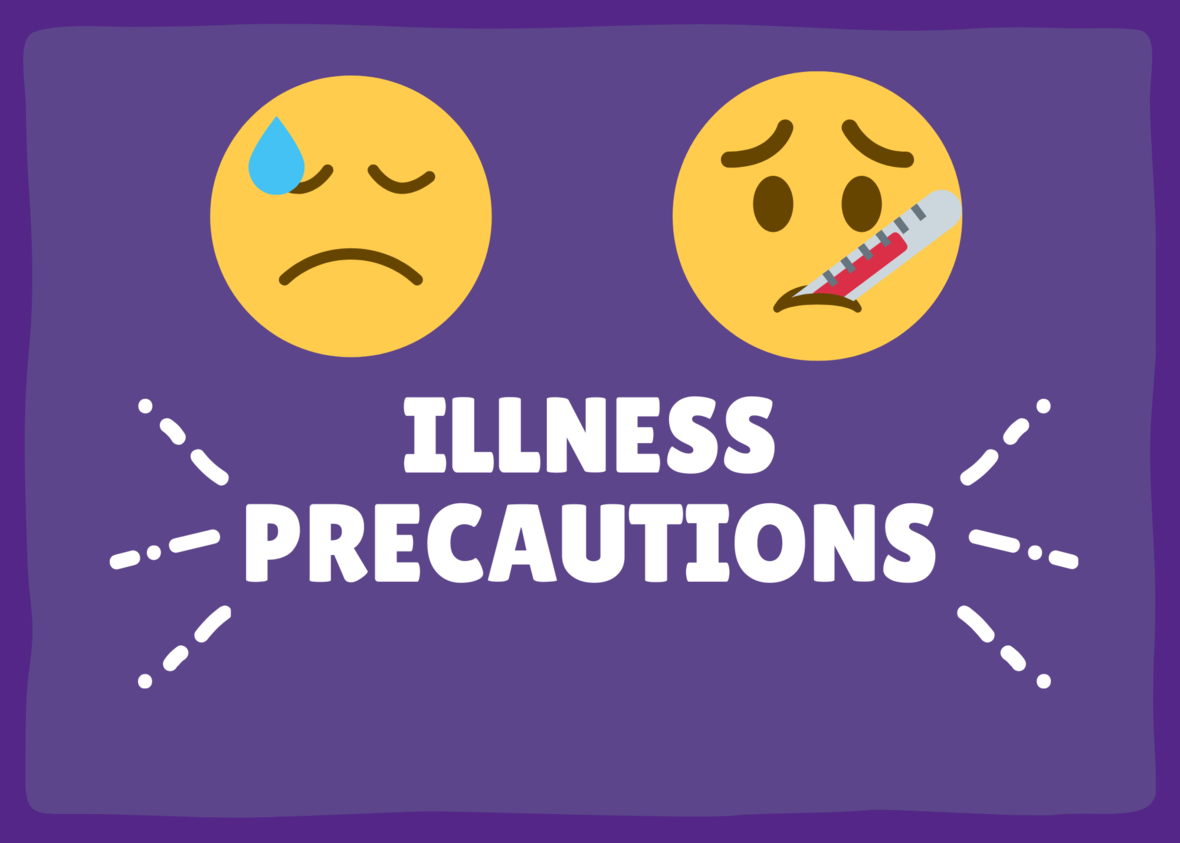# Illness Precautions

As we enter into cold and flu season, we need your help to mitigate the potential spread of illness by monitoring symptoms and keeping your child home if they present with a fever or are sick with other symptoms. Teachers and administrators will work with students to make up work in a timely manner once they are feeling better. By partnering with us, we will strive together to stay as healthy as possible during this season.

 table div table+table+table+table+table+table+table+table+table+table+table+table+table+table+table+table+table+table div table{width:100%;padding:0}table div table+table+table+table+table+table+table+table+table+table+table+table+table+table+table+table+table+table div table img{width:96.23%;padding:0;float:none}table div table+table+table+table+table+table+table+table+table+table+table+table+table+table+table+table+table+table div table td{width:100%;padding:0 1.88% 18px}/* styles */# The Lion's Den

The Lion's Den pop-up store will be at Friday night's game in Tibbetts this week for you to purchase some TKA spirit items.

▪ Friday, January 13, from 4:00 p.m. - 7:00 p.m. in Tibbetts during the basketball games.
 ▪ Friday, January 13, from 4:00 p.m. - 7:00 p.m. in Tibbetts during the basketball games.

Stop by and shop!

 table div table+table+table+table+table+table+table+table+table+table+table+table+table+table+table+table+table+table+table+table div table{width:100%;padding:0}table div table+table+table+table+table+table+table+table+table+table+table+table+table+table+table+table+table+table+table+table div table img{width:96.23%;padding:0;float:none}table div table+table+table+table+table+table+table+table+table+table+table+table+table+table+table+table+table+table+table+table div table td{width:100%;padding:0 1.88% 18px}/* styles */WHO: PreK3-5th grade students and their Dad (Grandfather, Uncle, or other important male figure)

WHEN: Saturday, March 4, from 6 p.m. to 8 p.m.

WHERE: Sevierville Civic Center (200 Gary R. Wade Blvd)

COST: \$80 per couple/\$40 per additional child (sorry, no refunds) Please make checks payable to TKA.

Forms and money can be turned into Mrs. Gioioso in the Elementary office. All forms and money are due by Wednesday, January 18. No late forms will be accepted.

For any questions regarding this event, please email Summer Delius at summerdelius@gmail.com.

 table div table+table+table+table+table+table+table+table+table+table+table+table+table+table+table+table+table+table+table+table+table+table+table div table{width:100%;padding:0}table div table+table+table+table+table+table+table+table+table+table+table+table+table+table+table+table+table+table+table+table+table+table+table div table img{width:96.23%;padding:0;float:none}table div table+table+table+table+table+table+table+table+table+table+table+table+table+table+table+table+table+table+table+table+table+table+table div table td{width:100%;padding:0 1.88% 18px}/* styles */# You're Invited!

## Friday, January 20

PTF is excited to share lunch with the PTF members at Pelanchos on Friday, January 20, at 11:00 a.m. We hope you will make plans to join us!

Not a member of PTF, don't worry, it's not too late to join. Use the link below to become a member of PTF.

 table div table+table+table+table+table+table+table+table+table+table+table+table+table+table+table+table+table+table+table+table+table+table+table+table+table+table div table{width:100%;padding:0}table div table+table+table+table+table+table+table+table+table+table+table+table+table+table+table+table+table+table+table+table+table+table+table+table+table+table div table img{width:96.23%;padding:0;float:none}table div table+table+table+table+table+table+table+table+table+table+table+table+table+table+table+table+table+table+table+table+table+table+table+table+table+table div table td{width:100%;padding:0 1.88% 18px}/* styles */# 2023 Love Gala

## Saturday, February 25

The 2nd Annual Love Gala is just around the corner! This semi-formal, adult only event is sure to be a wonderful evening filled with live entertainment, a silent auction, and a live auction.

Tickets for this event can be purchased by using the link below.

 table div table+table+table+table+table+table+table+table+table+table+table+table+table+table+table+table+table+table+table+table+table+table+table+table+table+table+table+table+table div table{width:100%;padding:0}table div table+table+table+table+table+table+table+table+table+table+table+table+table+table+table+table+table+table+table+table+table+table+table+table+table+table+table+table+table div table img{width:96.23%;padding:0;float:none}table div table+table+table+table+table+table+table+table+table+table+table+table+table+table+table+table+table+table+table+table+table+table+table+table+table+table+table+table+table div table td{width:100%;padding:0 1.88% 18px}/* styles */# Elementary Spring Clubs

TKA Elementary Families,

We are excited to announce that our TKA Elementary club spring opportunities will begin very soon. The spring section will begin the week of January 30th and end on April 21st.

Each club will include a \$20 participation fee to cover resources needed for club activities. Unfortunately, each club has a maximum number of 15 students.

Please click on a club to review the club description and registration link. Again, we will select the first participants until the maximum number is reached. We ask that you consider all options and register for clubs that fit your schedule for the year. To be fair to our club instructors, we ask that you choose a club where your child will be able to participate and attend 90% of the semester club activities.

All signup forms will close Friday, January 20th. Our club teachers will be in communication with participant families for their club area by Friday, January 27th. This communication will include the next steps prior to the first meeting the following week.

We are very blessed to have such wonderful teachers who are excited to provide these enriching opportunities after school hours to further enhance our elementary learning.1st-2nd Book Club will provide students with the opportunity to learn about authors/illustrators by engaging with their books deeply in a relaxed fun environment. Through group discussion and hands-on activities, students will practice age appropriate comprehension skills such as character analysis, distinguishing literary themes, understanding the elements of plot development, and using textual evidence. Book club will also provide time for readers to share book recommendations with each other, through book talks and book lists, in order to deepen their self-knowledge as a reader and broaden their range of choice in reading material. Reading choice is vital to developing a lifelong love of reading. Choice of author/illustrator will be voted on at the end of each club meeting so readers can have input and ownership of their experience.

3rd-5th Book Club will provide students with the opportunity to engage with texts deeply through a Battle of the Books style competition. Students will be given a wide selection of books to read from. Reading choice is vital to developing a lifelong love of reading. Students will be able to choose their own reading materials, within the parameters of the competition, giving them the opportunity to become more familiar with genres and writing styles that appeal to them. At each club meeting, students will compete on teams based on their book selections. Teams will work together to answer questions about the books read. Through this gaming experience, students will have the opportunity to practice comprehension skills such as character analysis, distinguishing literary themes, understanding the elements of plot development, and using textual evidence.Bible Club will include Bible Drill, Memory Verses, Bible Story Interacting (drawing, drama, games, questions, songs,) . . . and Developing a Personal Quiet Time.# K4-K5 STEAM Club

K4-K5 STEAM Club will include a variety of hands-on activities focusing on science, technology, engineering, arts, and mathematics that are age appropriate for our little lions.# STEAM Club (1-2 grades only)

1st-2nd Grade STEAM Club will include a variety of hands-on activities focusing on science, technology, engineering, arts, and mathematics that are age appropriate for our little lions.# Whiz Kidz (3-5 grades only)

This STEAM Club, "Whiz Kidz", will include a variety of hands-on activities focusing on science, technology, engineering, arts, and mathematics that are age appropriate for our intermediate lions.

 table div table+table+table+table+table+table+table+table+table+table+table+table+table+table+table+table+table+table+table+table+table+table+table+table+table+table+table+table+table+table+table+table+table+table+table+table+table+table+table+table+table+table+table div table{width:100%;padding:0}table div table+table+table+table+table+table+table+table+table+table+table+table+table+table+table+table+table+table+table+table+table+table+table+table+table+table+table+table+table+table+table+table+table+table+table+table+table+table+table+table+table+table+table div table img{width:96.23%;padding:0;float:none}table div table+table+table+table+table+table+table+table+table+table+table+table+table+table+table+table+table+table+table+table+table+table+table+table+table+table+table+table+table+table+table+table+table+table+table+table+table+table+table+table+table+table+table div table td{width:100%;padding:0 1.88% 18px}/* styles */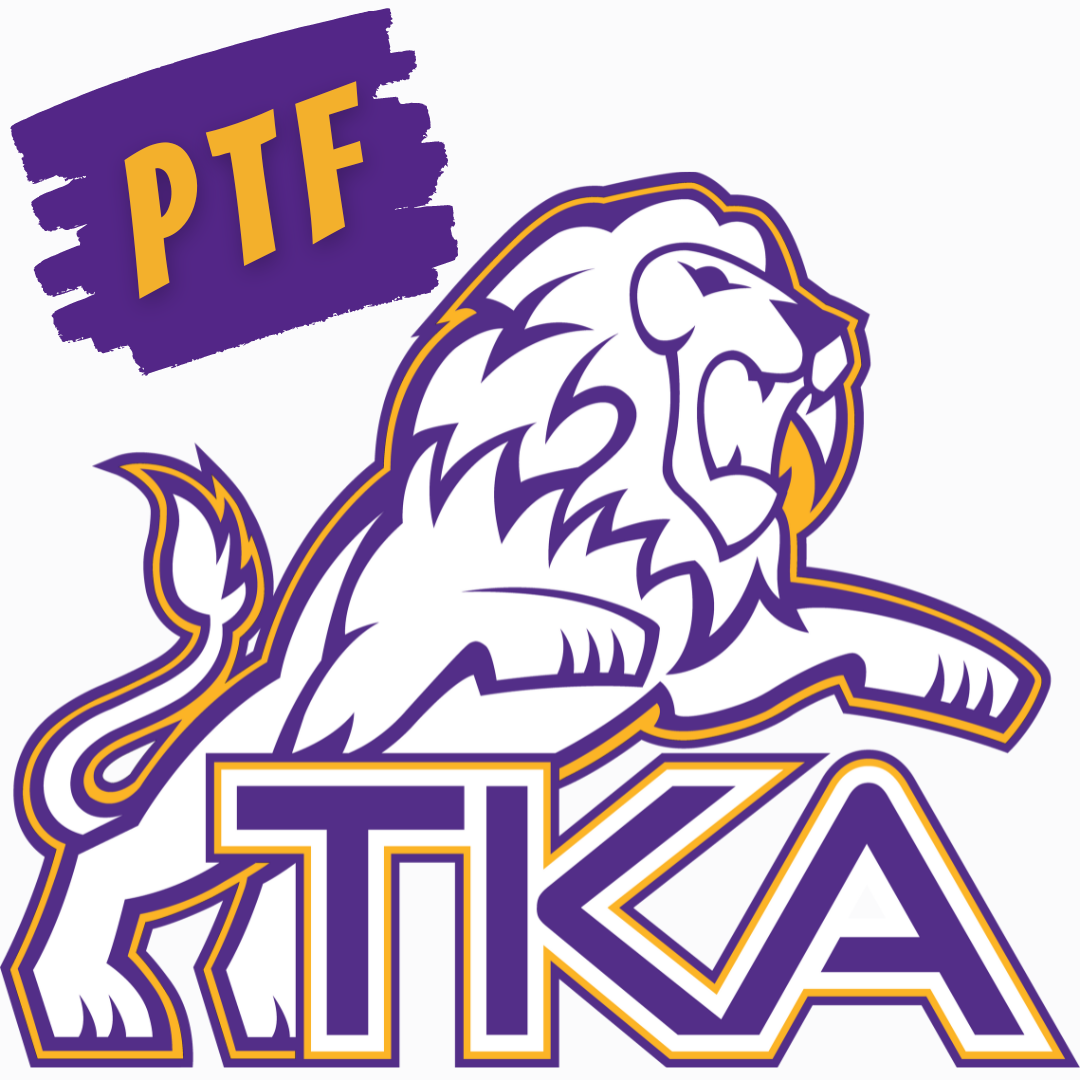# PTF Highlights

Thanks to our Parent Teacher Fellowship (PTF) and your generous support, some amazing things have taken place on campus for our students and teachers.

Take a moment and read the accomplishments that have taken place through our PTF!

## Recess Relief for PreK Teachers

Once a month, PTF provides recess relief for our PreK teachers by watching the students while they play and allowing the teachers to eat lunch and have a small break.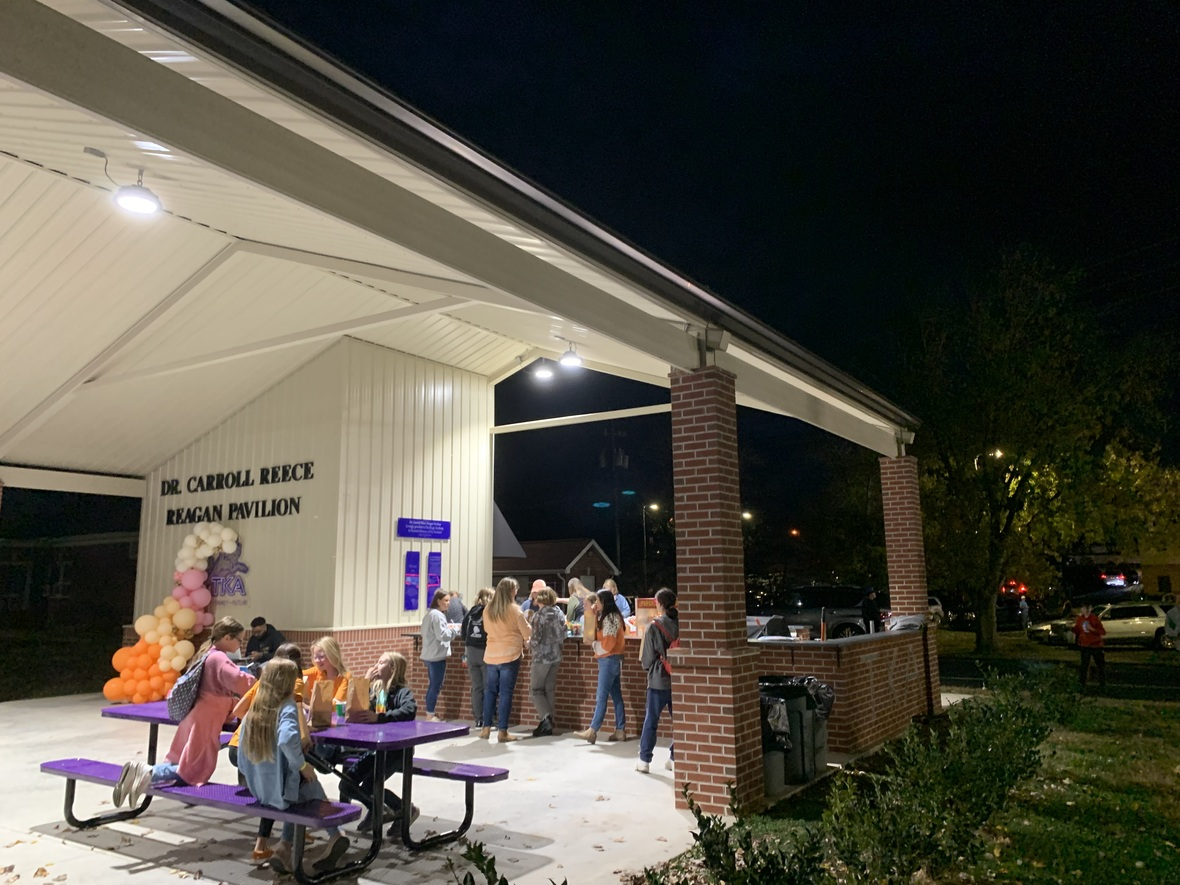## Middle School Breakaway

PTF sponsored and staffed a middle school breakaway for our event.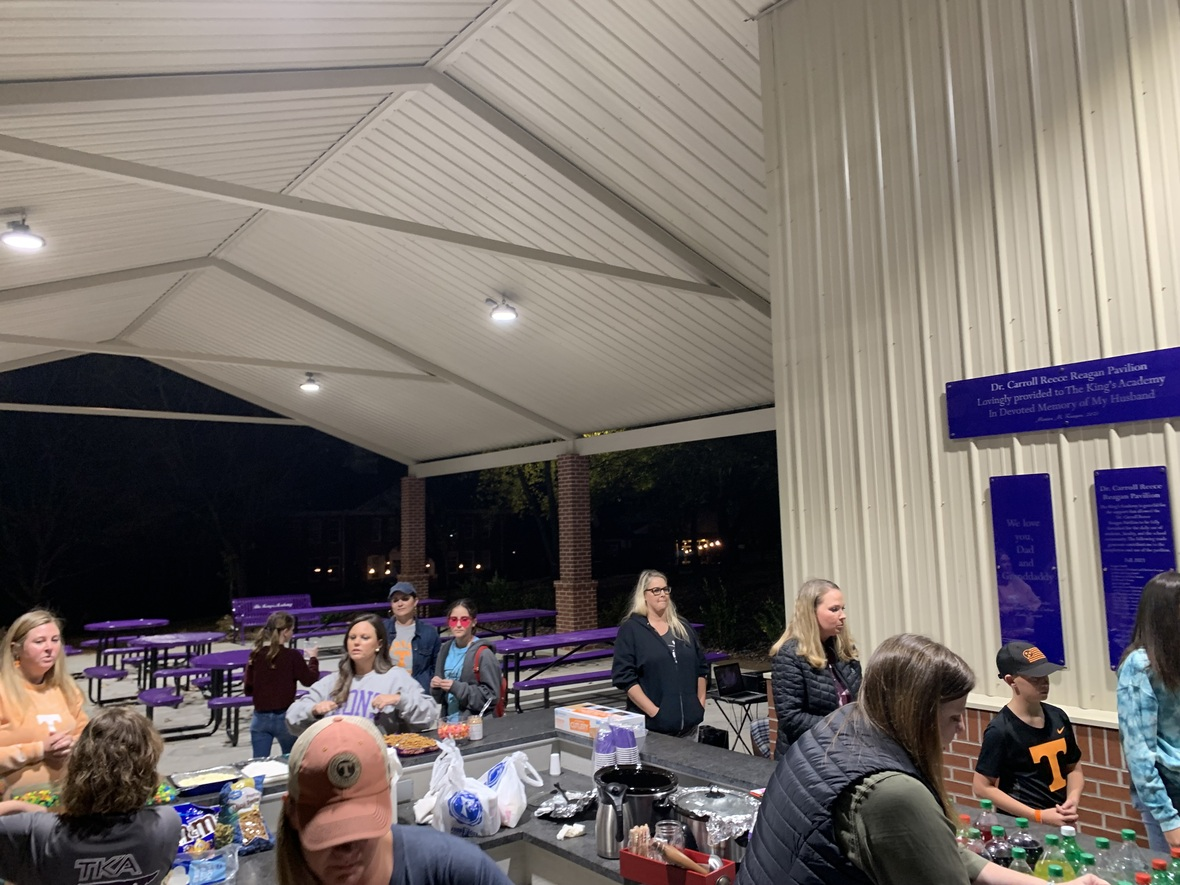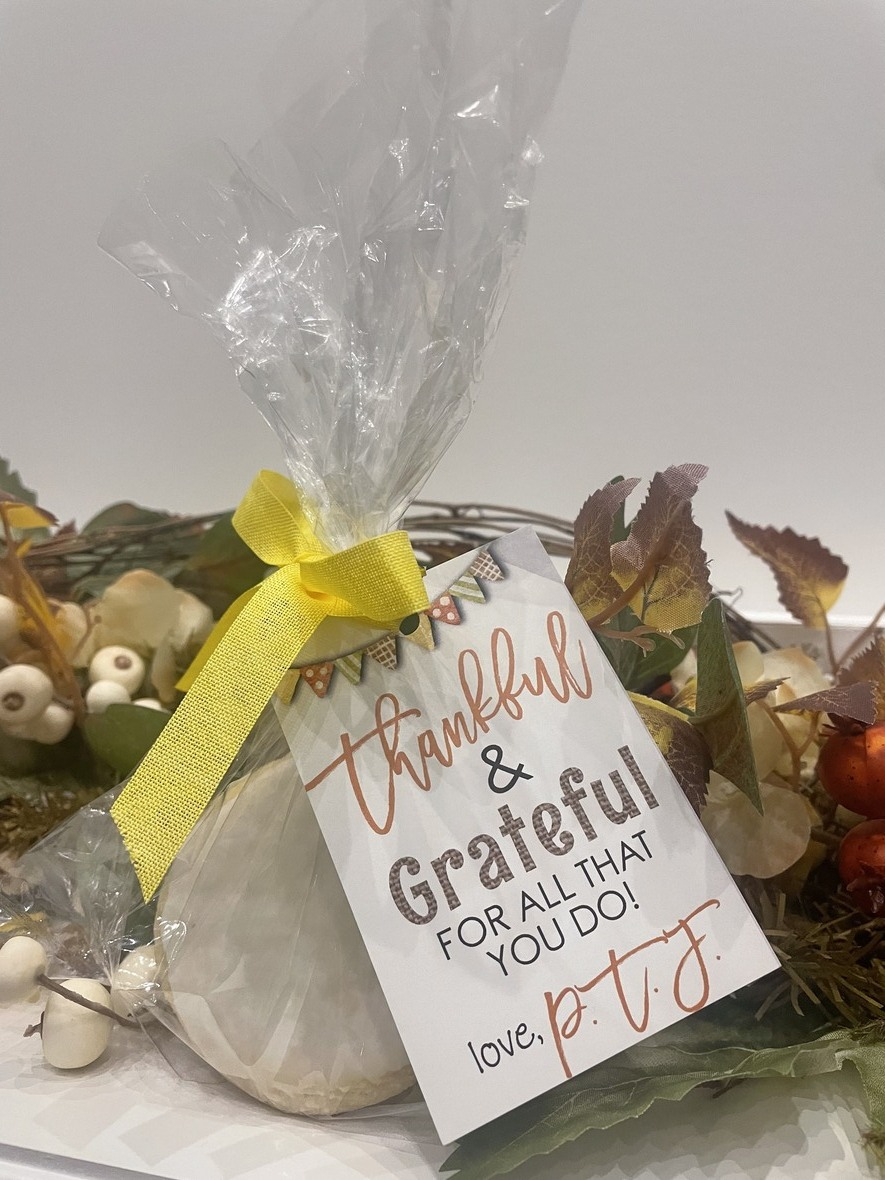## Faculty and Staff Cookies for Thanksgiving

PTF continuously supplies the faculty and staff with delicious snacks and drinks throughout the year.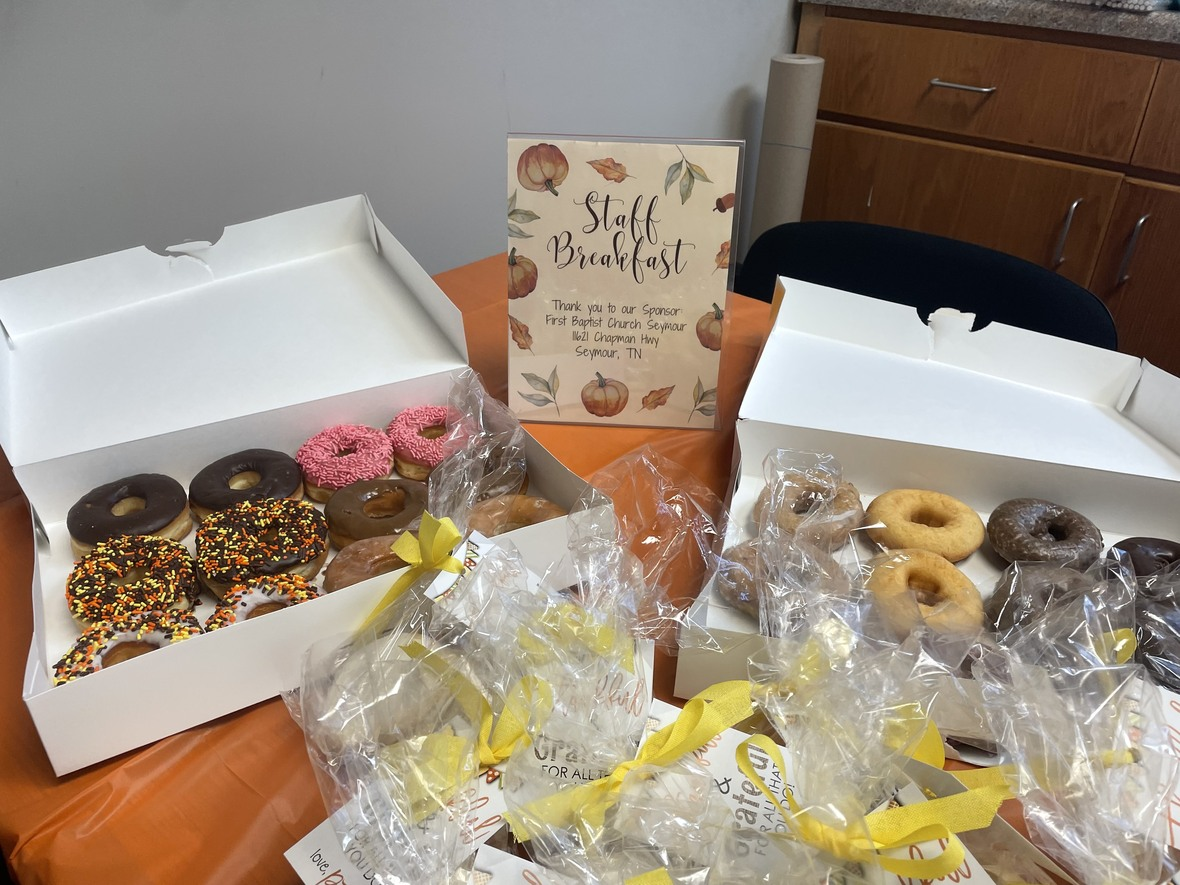## PTF Faculty and Staff Breakfast Partnerships

PTF continuously supplies the faculty and staff with delicious snacks and drinks throughout the year with the partnership of various churches and businesses.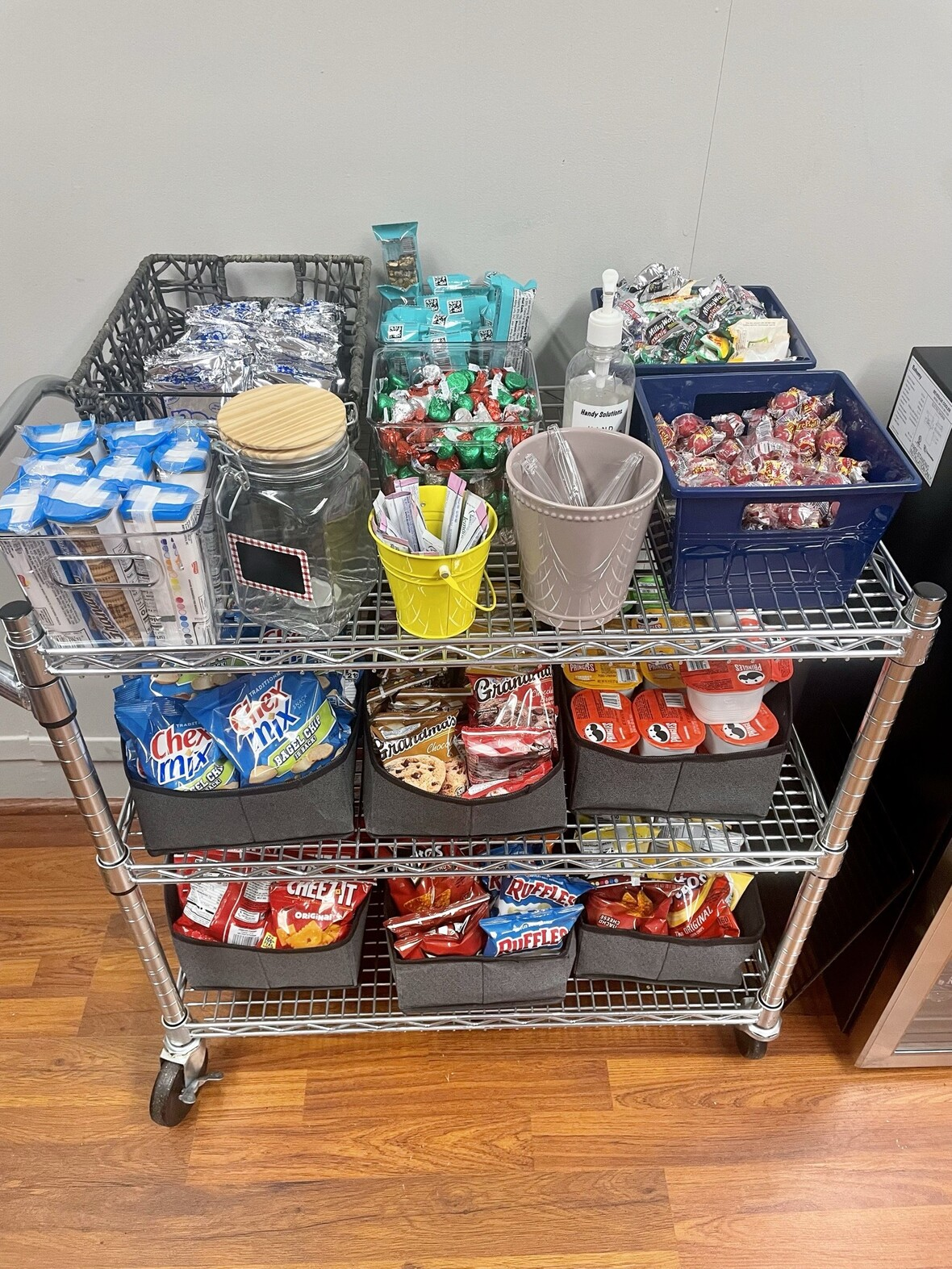## Faculty and Staff Snacks

PTF continuously supplies the faculty and staff with delicious snacks and drinks throughout the year.## Elf Closet

PTF hosted the annual Elf Closet for our Little Lions to shop for friends and family during the Christmas season. PTF volunteers also wrapped and labeled gifts for our Lions. Thank you to all that shopped and volunteered!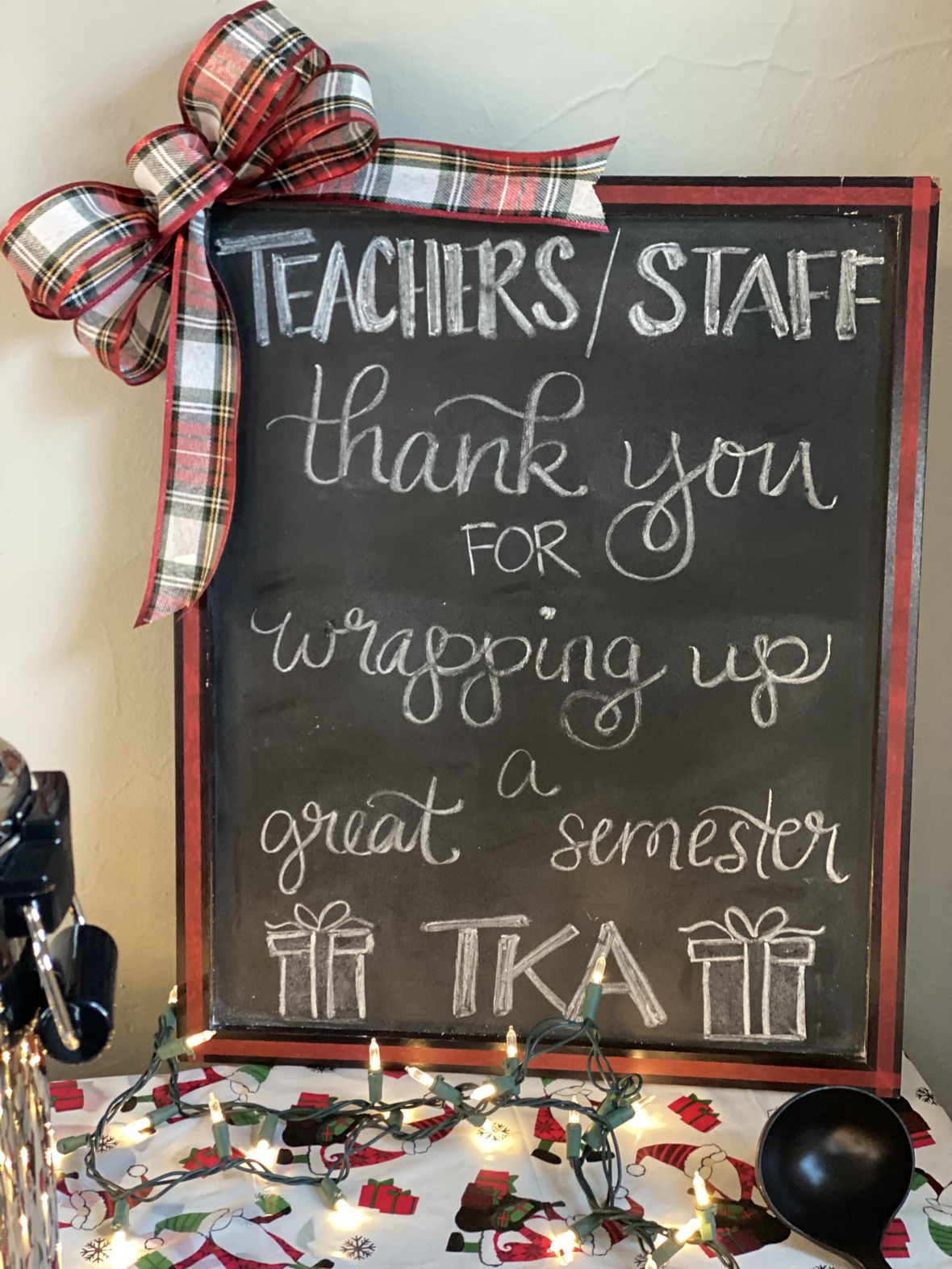## Faculty and Staff Gift Wrapping

This event has quickly become a staff favorite! PTF volunteers provide snacks and wrap faculty and staff gifts for their families. Teachers drop off their gifts, fill out gift tags, enjoy snacks, and return when the gifts are wrapped.PTF provided a gift card to each of our teachers for Christmas.

 /* styles */ Thank you to our PTF for sponsoring these many events and activities! Also, thank you to all who have participated in these events and activities to help make them a success. If you have not joined our PTF yet, it's not too late! Use this link below to sign up to be a member of PTF. The next PTF meeting will take TOMORROW, at 3:15 p.m. in Brandes.
 table div table+table+table+table+table+table+table+table+table+table+table+table+table+table+table+table+table+table+table+table+table+table+table+table+table+table+table+table+table+table+table+table+table+table+table+table+table+table+table+table+table+table+table+table+table+table+table+table+table+table+table+table+table+table+table+table div table{width:100%;padding:0}table div table+table+table+table+table+table+table+table+table+table+table+table+table+table+table+table+table+table+table+table+table+table+table+table+table+table+table+table+table+table+table+table+table+table+table+table+table+table+table+table+table+table+table+table+table+table+table+table+table+table+table+table+table+table+table+table div table img{width:96.23%;padding:0;float:none}table div table+table+table+table+table+table+table+table+table+table+table+table+table+table+table+table+table+table+table+table+table+table+table+table+table+table+table+table+table+table+table+table+table+table+table+table+table+table+table+table+table+table+table+table+table+table+table+table+table+table+table+table+table+table+table+table div table td{width:100%;padding:0 1.88% 18px}/* styles */table div table+table+table+table+table+table+table+table+table+table+table+table+table+table+table+table+table+table+table+table+table+table+table+table+table+table+table+table+table+table+table+table+table+table+table+table+table+table+table+table+table+table+table+table+table+table+table+table+table+table+table+table+table+table+table+table+table+table div table{width:100%;padding:0}table div table+table+table+table+table+table+table+table+table+table+table+table+table+table+table+table+table+table+table+table+table+table+table+table+table+table+table+table+table+table+table+table+table+table+table+table+table+table+table+table+table+table+table+table+table+table+table+table+table+table+table+table+table+table+table+table+table+table div table img{width:96.23%;padding:0;float:none}table div table+table+table+table+table+table+table+table+table+table+table+table+table+table+table+table+table+table+table+table+table+table+table+table+table+table+table+table+table+table+table+table+table+table+table+table+table+table+table+table+table+table+table+table+table+table+table+table+table+table+table+table+table+table+table+table+table+table div table td{width:100%;padding:0 1.88% 18px}/* styles */# We're Hiring!

TKA has an employment opportunity available in food services.

Food Services Employee: TKA is seeking an employee to faithfully serve in our food services department. Prior food service experience is preferred. Please email resumes to HR@thekingsacademy.net or call 865-573-8321.

 table div table+table+table+table+table+table+table+table+table+table+table+table+table+table+table+table+table+table+table+table+table+table+table+table+table+table+table+table+table+table+table+table+table+table+table+table+table+table+table+table+table+table+table+table+table+table+table+table+table+table+table+table+table+table+table+table+table+table+table+table+table div table{width:100%;padding:0}table div table+table+table+table+table+table+table+table+table+table+table+table+table+table+table+table+table+table+table+table+table+table+table+table+table+table+table+table+table+table+table+table+table+table+table+table+table+table+table+table+table+table+table+table+table+table+table+table+table+table+table+table+table+table+table+table+table+table+table+table+table div table img{width:96.23%;padding:0;float:none}table div table+table+table+table+table+table+table+table+table+table+table+table+table+table+table+table+table+table+table+table+table+table+table+table+table+table+table+table+table+table+table+table+table+table+table+table+table+table+table+table+table+table+table+table+table+table+table+table+table+table+table+table+table+table+table+table+table+table+table+table+table div table td{width:100%;padding:0 1.88% 18px}/* styles */# D1 Training

I hope the holiday season has been wonderful for you all. I am happy to announce that we will be adding morning workout times in the near future. The times added will be a 6 am and 7 am class for adults. We understand time is important, and we want to make sure that we help and assist you as much as we can in order for you to reach your health goals. Many times resolutions fail because of a lack of accountability- That's why D1 is here! To keep you accountable to yourself, your family, and your commitment. Join the team!

Melvin Jones
D1 Sevierville
865-719-9421table div table+table+table+table+table+table+table+table+table+table+table+table+table+table+table+table+table+table+table+table+table+table+table+table+table+table+table+table+table+table+table+table+table+table+table+table+table+table+table+table+table+table+table+table+table+table+table+table+table+table+table+table+table+table+table+table+table+table+table+table+table+table+table+table div table{width:100%;padding:0}table div table+table+table+table+table+table+table+table+table+table+table+table+table+table+table+table+table+table+table+table+table+table+table+table+table+table+table+table+table+table+table+table+table+table+table+table+table+table+table+table+table+table+table+table+table+table+table+table+table+table+table+table+table+table+table+table+table+table+table+table+table+table+table+table div table img{width:96.23%;padding:0;float:none}table div table+table+table+table+table+table+table+table+table+table+table+table+table+table+table+table+table+table+table+table+table+table+table+table+table+table+table+table+table+table+table+table+table+table+table+table+table+table+table+table+table+table+table+table+table+table+table+table+table+table+table+table+table+table+table+table+table+table+table+table+table+table+table+table div table td{width:100%;padding:0 1.88% 18px}/* styles */# 2022-23 Yearbooks

2022-23 yearbooks are now on sale! Scan this QR code to visit the site to purchase yours today. For any questions regarding yearbooks, contact Mr. Josh Smith at jsmith@thekingsacademy.net.

 table div table+table+table+table+table+table+table+table+table+table+table+table+table+table+table+table+table+table+table+table+table+table+table+table+table+table+table+table+table+table+table+table+table+table+table+table+table+table+table+table+table+table+table+table+table+table+table+table+table+table+table+table+table+table+table+table+table+table+table+table+table+table+table+table+table+table div table{width:100%;padding:0}table div table+table+table+table+table+table+table+table+table+table+table+table+table+table+table+table+table+table+table+table+table+table+table+table+table+table+table+table+table+table+table+table+table+table+table+table+table+table+table+table+table+table+table+table+table+table+table+table+table+table+table+table+table+table+table+table+table+table+table+table+table+table+table+table+table+table div table img{width:96.23%;padding:0;float:none}table div table+table+table+table+table+table+table+table+table+table+table+table+table+table+table+table+table+table+table+table+table+table+table+table+table+table+table+table+table+table+table+table+table+table+table+table+table+table+table+table+table+table+table+table+table+table+table+table+table+table+table+table+table+table+table+table+table+table+table+table+table+table+table+table+table+table div table td{width:100%;padding:0 1.88% 18px}/* styles */# 9-12th Spring Break Trip: France, Germany and The Alps

## March 11-18, 2023

 table div table+table+table+table+table+table+table+table+table+table+table+table+table+table+table+table+table+table+table+table+table+table+table+table+table+table+table+table+table+table+table+table+table+table+table+table+table+table+table+table+table+table+table+table+table+table+table+table+table+table+table+table+table+table+table+table+table+table+table+table+table+table+table+table+table+table+table+table div table{width:100%;padding:0}table div table+table+table+table+table+table+table+table+table+table+table+table+table+table+table+table+table+table+table+table+table+table+table+table+table+table+table+table+table+table+table+table+table+table+table+table+table+table+table+table+table+table+table+table+table+table+table+table+table+table+table+table+table+table+table+table+table+table+table+table+table+table+table+table+table+table+table+table div table img{width:96.23%;padding:0;float:none}table div table+table+table+table+table+table+table+table+table+table+table+table+table+table+table+table+table+table+table+table+table+table+table+table+table+table+table+table+table+table+table+table+table+table+table+table+table+table+table+table+table+table+table+table+table+table+table+table+table+table+table+table+table+table+table+table+table+table+table+table+table+table+table+table+table+table+table+table div table td{width:100%;padding:0 1.88% 18px}/* styles */# Weekly Sports Schedule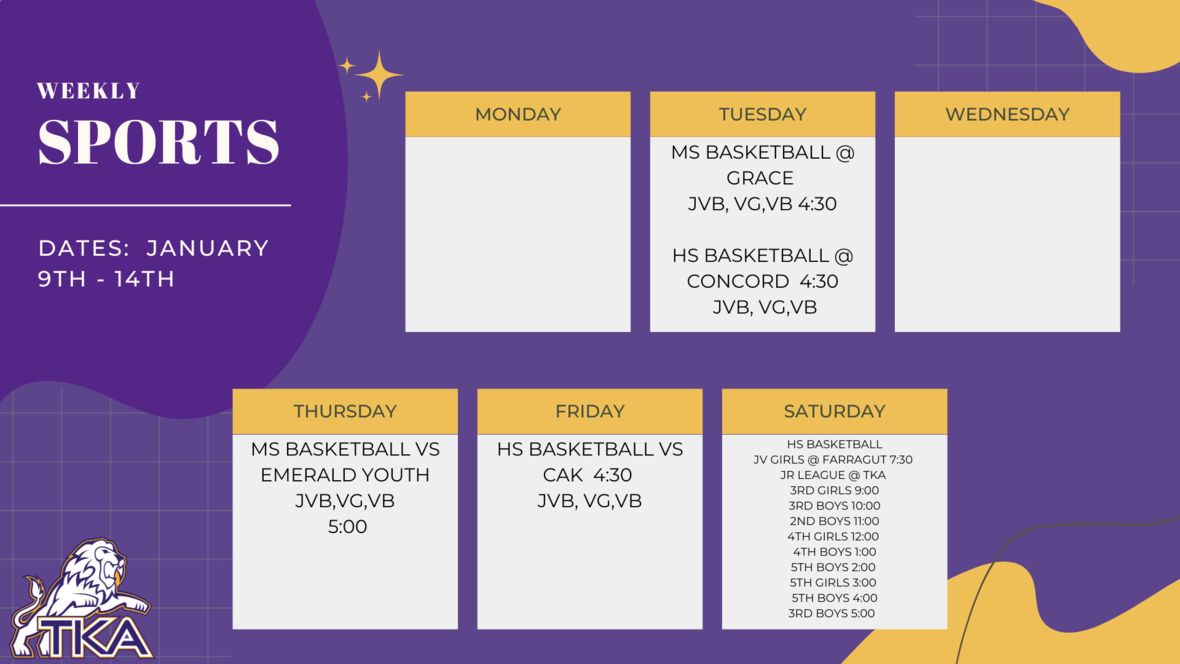table div table+table+table+table+table+table+table+table+table+table+table+table+table+table+table+table+table+table+table+table+table+table+table+table+table+table+table+table+table+table+table+table+table+table+table+table+table+table+table+table+table+table+table+table+table+table+table+table+table+table+table+table+table+table+table+table+table+table+table+table+table+table+table+table+table+table+table+table+table+table+table div table{width:100%;padding:0}table div table+table+table+table+table+table+table+table+table+table+table+table+table+table+table+table+table+table+table+table+table+table+table+table+table+table+table+table+table+table+table+table+table+table+table+table+table+table+table+table+table+table+table+table+table+table+table+table+table+table+table+table+table+table+table+table+table+table+table+table+table+table+table+table+table+table+table+table+table+table+table div table img{width:96.23%;padding:0;float:none}table div table+table+table+table+table+table+table+table+table+table+table+table+table+table+table+table+table+table+table+table+table+table+table+table+table+table+table+table+table+table+table+table+table+table+table+table+table+table+table+table+table+table+table+table+table+table+table+table+table+table+table+table+table+table+table+table+table+table+table+table+table+table+table+table+table+table+table+table+table+table+table div table td{width:100%;padding:0 1.88% 18px}/* styles */# Important information for the 2022-2023 School Year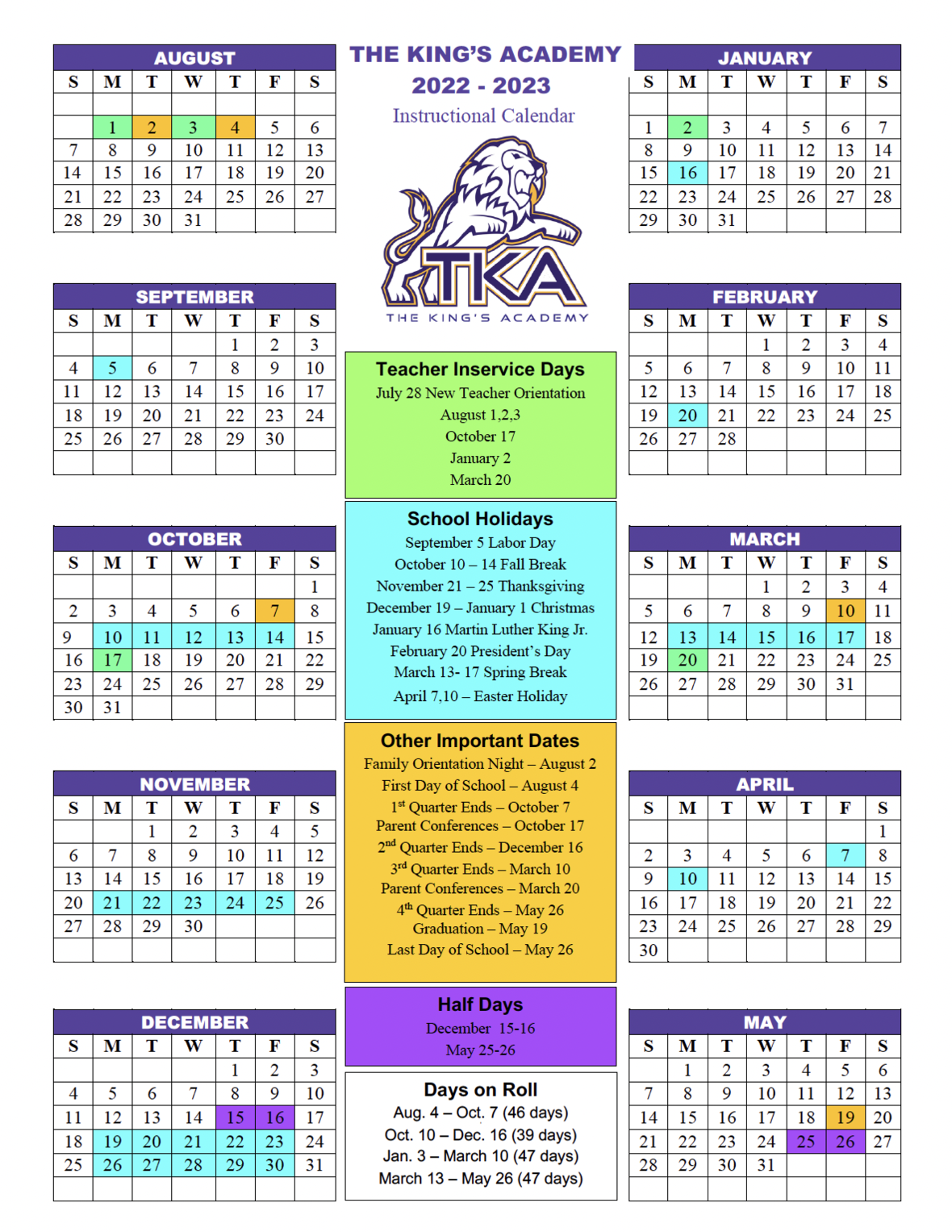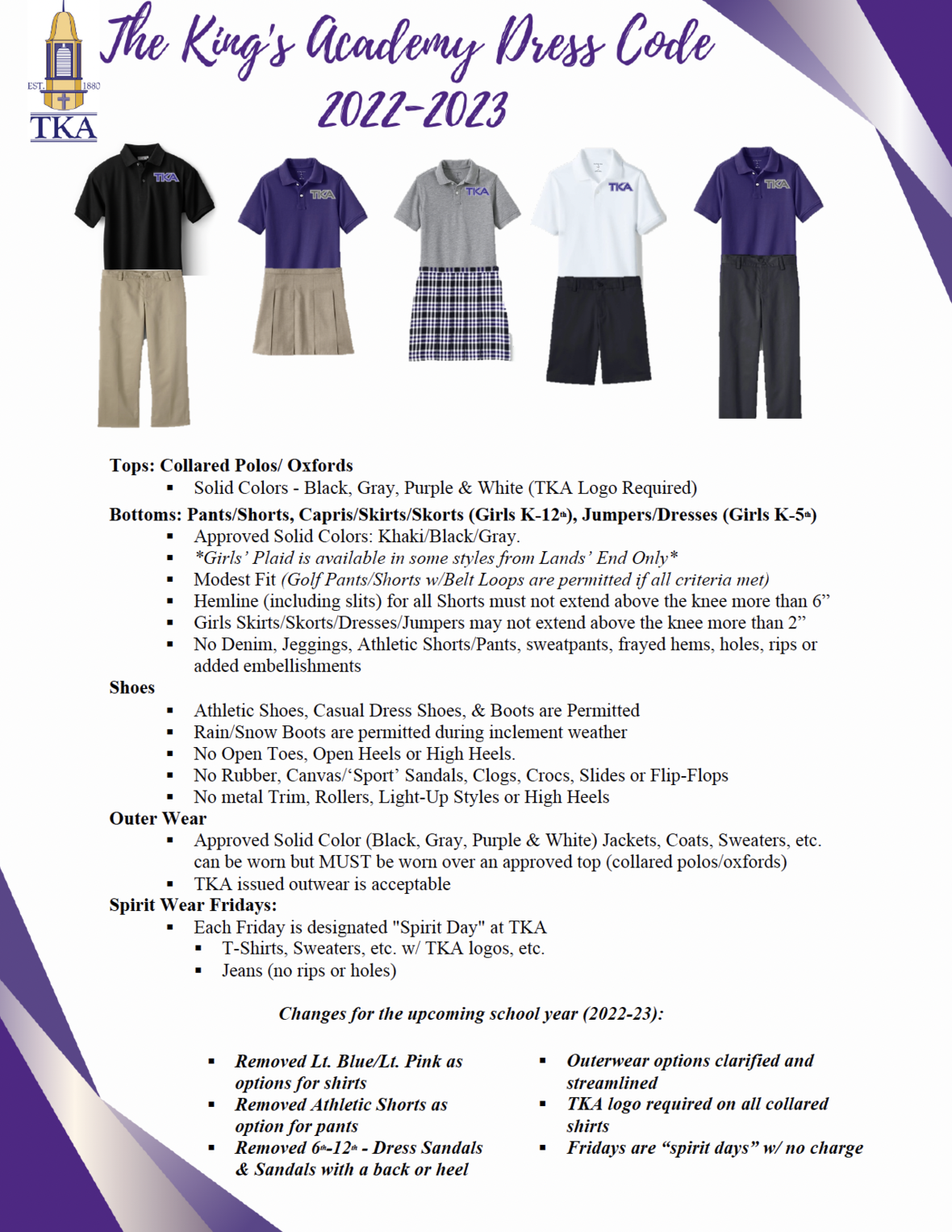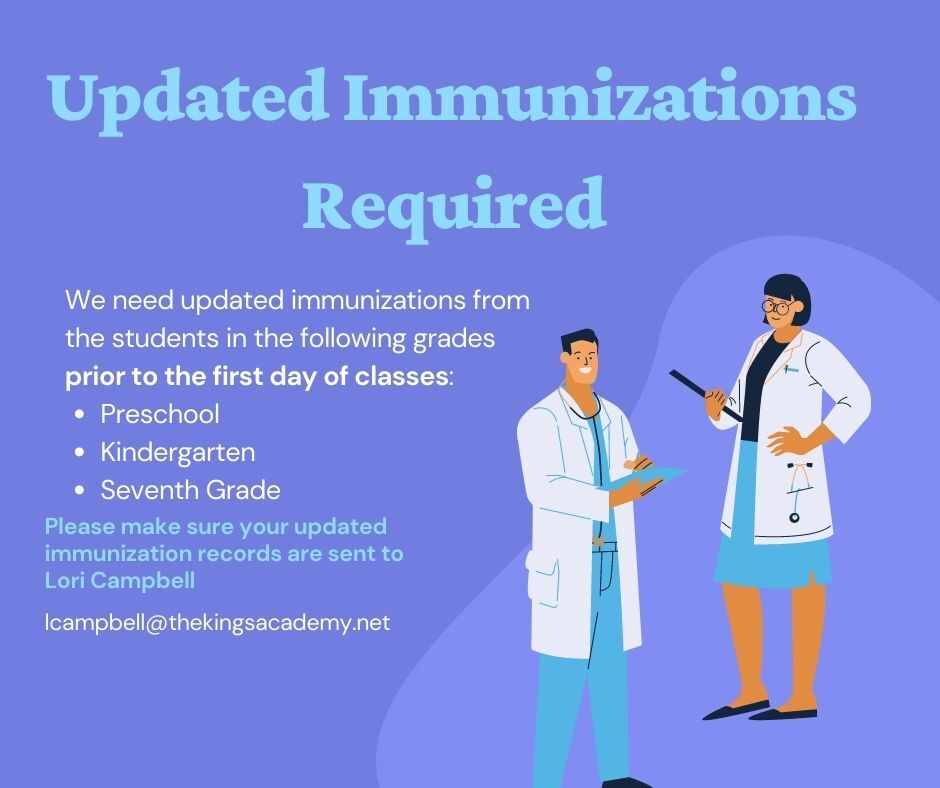table div table+table+table+table+table+table+table+table+table+table+table+table+table+table+table+table+table+table+table+table+table+table+table+table+table+table+table+table+table+table+table+table+table+table+table+table+table+table+table+table+table+table+table+table+table+table+table+table+table+table+table+table+table+table+table+table+table+table+table+table+table+table+table+table+table+table+table+table+table+table+table+table+table+table+table+table div table{width:100%;padding:0}table div table+table+table+table+table+table+table+table+table+table+table+table+table+table+table+table+table+table+table+table+table+table+table+table+table+table+table+table+table+table+table+table+table+table+table+table+table+table+table+table+table+table+table+table+table+table+table+table+table+table+table+table+table+table+table+table+table+table+table+table+table+table+table+table+table+table+table+table+table+table+table+table+table+table+table+table div table img{width:96.23%;padding:0;float:none}table div table+table+table+table+table+table+table+table+table+table+table+table+table+table+table+table+table+table+table+table+table+table+table+table+table+table+table+table+table+table+table+table+table+table+table+table+table+table+table+table+table+table+table+table+table+table+table+table+table+table+table+table+table+table+table+table+table+table+table+table+table+table+table+table+table+table+table+table+table+table+table+table+table+table+table+table div table td{width:100%;padding:0 1.88% 18px}/* styles */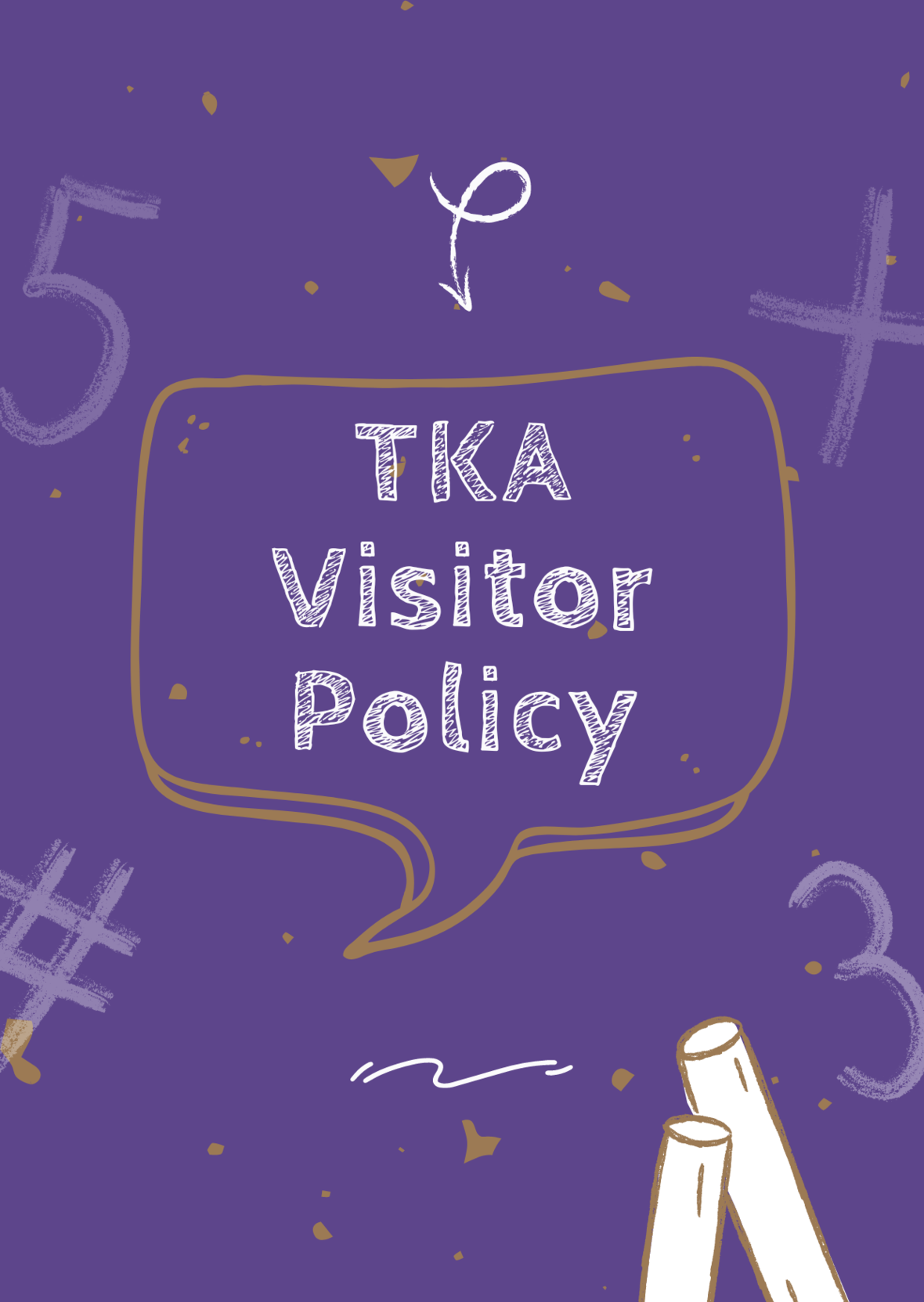# TKA Visitor Policy

In order to help maintain a safe and instructionally based learning environment for our students, all visitors for middle and high school must enter through the front door of the Anderson Building and sign in in the middle/high school office in order to receive a visitor's pass. All visitors for elementary must enter the front door of the Ogle Building in order to receive a visitor's pass. This pass should be visible at all times so that TKA faculty and staff know that you have checked in at the office.

Our doors are open to visitors, but please call ahead of time to schedule conferences with your child’s teachers. This will prevent interruptions to instruction and the loss of the valuable learning time of our students. Adults will not be allowed to wander the halls of the building or hold conferences with teachers during instructional times.

TKA family lunch visitors should follow the above procedure. Lunch tickets are \$5 each.

Students who are not enrolled at TKA are not permitted to visit TKA students during the school day, which includes lunch.

This policy has been developed in order to help maintain a safe and instruction focused environment at school each day. Any visitor who does not meet these expectations may be asked to leave school grounds.

 table div table+table+table+table+table+table+table+table+table+table+table+table+table+table+table+table+table+table+table+table+table+table+table+table+table+table+table+table+table+table+table+table+table+table+table+table+table+table+table+table+table+table+table+table+table+table+table+table+table+table+table+table+table+table+table+table+table+table+table+table+table+table+table+table+table+table+table+table+table+table+table+table+table+table+table+table+table+table div table{width:100%;padding:0}table div table+table+table+table+table+table+table+table+table+table+table+table+table+table+table+table+table+table+table+table+table+table+table+table+table+table+table+table+table+table+table+table+table+table+table+table+table+table+table+table+table+table+table+table+table+table+table+table+table+table+table+table+table+table+table+table+table+table+table+table+table+table+table+table+table+table+table+table+table+table+table+table+table+table+table+table+table+table div table img{width:96.23%;padding:0;float:none}table div table+table+table+table+table+table+table+table+table+table+table+table+table+table+table+table+table+table+table+table+table+table+table+table+table+table+table+table+table+table+table+table+table+table+table+table+table+table+table+table+table+table+table+table+table+table+table+table+table+table+table+table+table+table+table+table+table+table+table+table+table+table+table+table+table+table+table+table+table+table+table+table+table+table+table+table+table+table div table td{width:100%;padding:0 1.88% 18px}/* styles *//* styles */
 table div table+table+table+table+table+table+table+table+table+table+table+table+table+table+table+table+table+table+table+table+table+table+table+table+table+table+table+table+table+table+table+table+table+table+table+table+table+table+table+table+table+table+table+table+table+table+table+table+table+table+table+table+table+table+table+table+table+table+table+table+table+table+table+table+table+table+table+table+table+table+table+table+table+table+table+table+table+table+table+table+table div table{width:100%;padding:0}table div table+table+table+table+table+table+table+table+table+table+table+table+table+table+table+table+table+table+table+table+table+table+table+table+table+table+table+table+table+table+table+table+table+table+table+table+table+table+table+table+table+table+table+table+table+table+table+table+table+table+table+table+table+table+table+table+table+table+table+table+table+table+table+table+table+table+table+table+table+table+table+table+table+table+table+table+table+table+table+table+table div table img{width:96.23%;padding:0;float:none}table div table+table+table+table+table+table+table+table+table+table+table+table+table+table+table+table+table+table+table+table+table+table+table+table+table+table+table+table+table+table+table+table+table+table+table+table+table+table+table+table+table+table+table+table+table+table+table+table+table+table+table+table+table+table+table+table+table+table+table+table+table+table+table+table+table+table+table+table+table+table+table+table+table+table+table+table+table+table+table+table+table div table td{width:100%;padding:0 1.88% 18px}/* styles */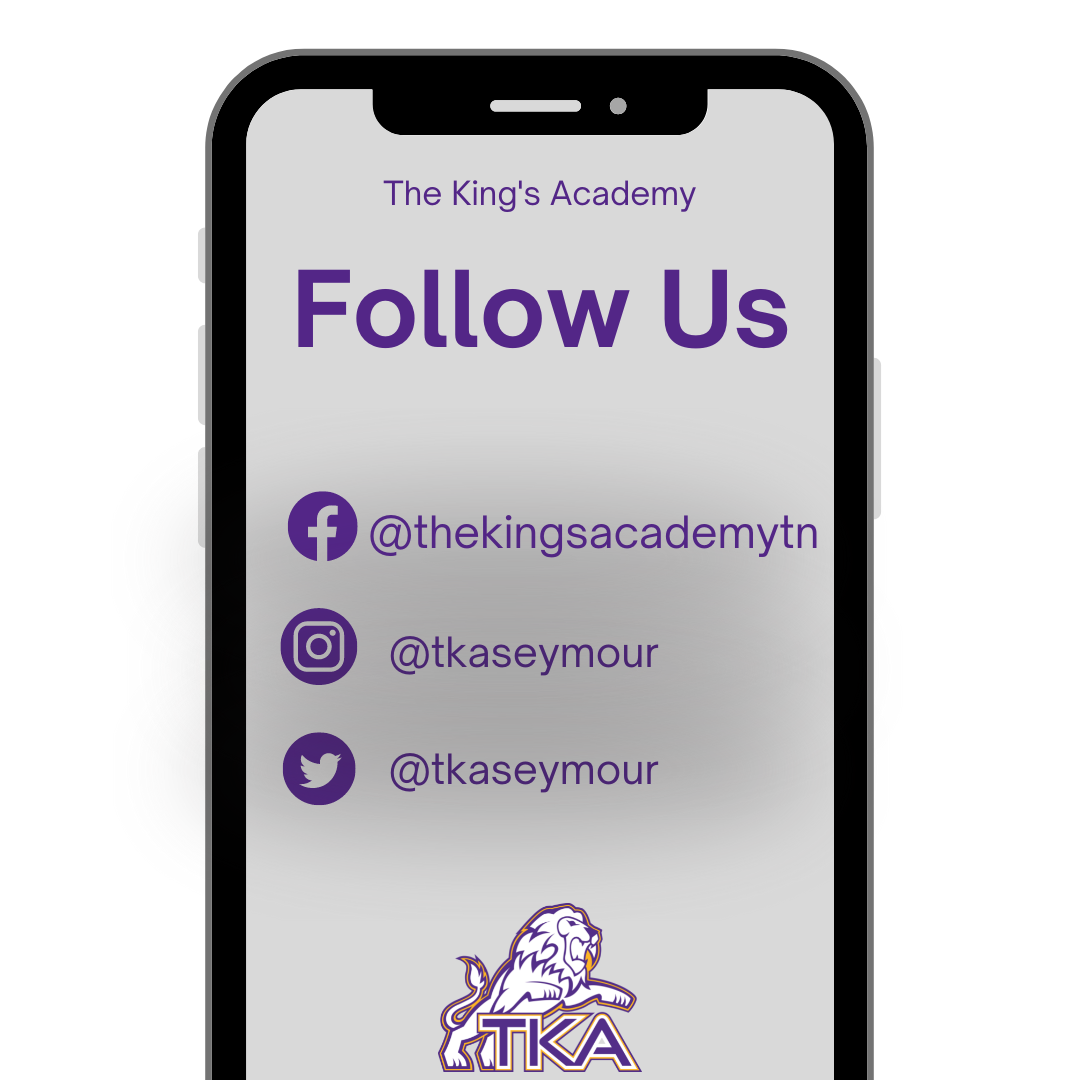table div table+table+table+table+table+table+table+table+table+table+table+table+table+table+table+table+table+table+table+table+table+table+table+table+table+table+table+table+table+table+table+table+table+table+table+table+table+table+table+table+table+table+table+table+table+table+table+table+table+table+table+table+table+table+table+table+table+table+table+table+table+table+table+table+table+table+table+table+table+table+table+table+table+table+table+table+table+table+table+table+table+table+table div table{width:100%;padding:0}table div table+table+table+table+table+table+table+table+table+table+table+table+table+table+table+table+table+table+table+table+table+table+table+table+table+table+table+table+table+table+table+table+table+table+table+table+table+table+table+table+table+table+table+table+table+table+table+table+table+table+table+table+table+table+table+table+table+table+table+table+table+table+table+table+table+table+table+table+table+table+table+table+table+table+table+table+table+table+table+table+table+table+table div table img{width:96.23%;padding:0;float:none}table div table+table+table+table+table+table+table+table+table+table+table+table+table+table+table+table+table+table+table+table+table+table+table+table+table+table+table+table+table+table+table+table+table+table+table+table+table+table+table+table+table+table+table+table+table+table+table+table+table+table+table+table+table+table+table+table+table+table+table+table+table+table+table+table+table+table+table+table+table+table+table+table+table+table+table+table+table+table+table+table+table+table+table div table td{width:100%;padding:0 1.88% 18px}/* styles *//* styles */ The King's Academy
 Like   Tweet